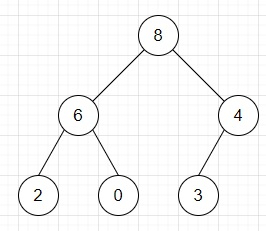# Program to check heap is forming max heap or not in Python

Suppose we have a list representing a heap tree. As we know heap is a complete binary tree. We have to check whether the elements are forming max heap or not. As we know for max heap every element is larger than both of its children.

So, if the input is like nums = [8, 6, 4, 2, 0, 3], then the output will be True because, all elements are larger than their children.To solve this, we will follow these steps −

• n := size of nums
• for i in range 0 to n - 1, do
• m := i * 2
• num := nums[i]
• if m + 1 < n, then
• if num < nums[m + 1], then
• return False
• if m + 2 < n, then
• if num < nums[m + 2], then
• return False
• return True

## Example

Let us see the following implementation to get better understanding −

def solve(nums):
n = len(nums)
for i in range(n):
m = i * 2
num = nums[i]
if m + 1 < n:
if num < nums[m + 1]:
return False
if m + 2 < n:
if num < nums[m + 2]:
return False
return True

nums = [8, 6, 4, 2, 0, 3]
print(solve(nums))

## Input

[8, 6, 4, 2, 0, 3]

## Output

True